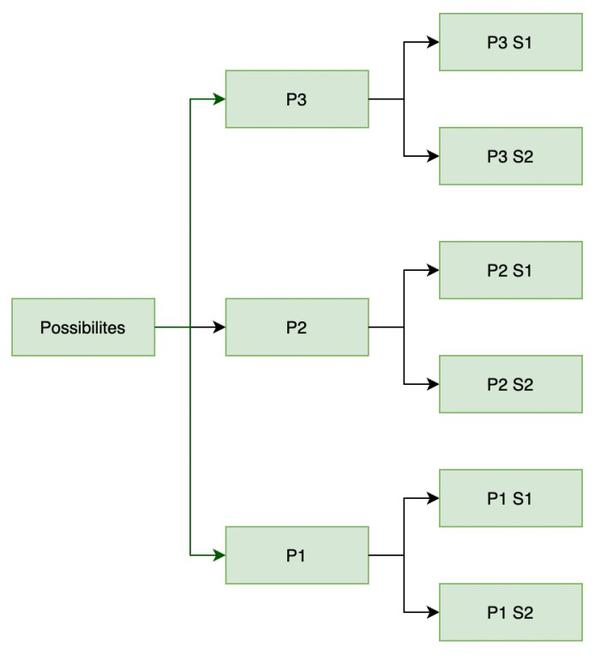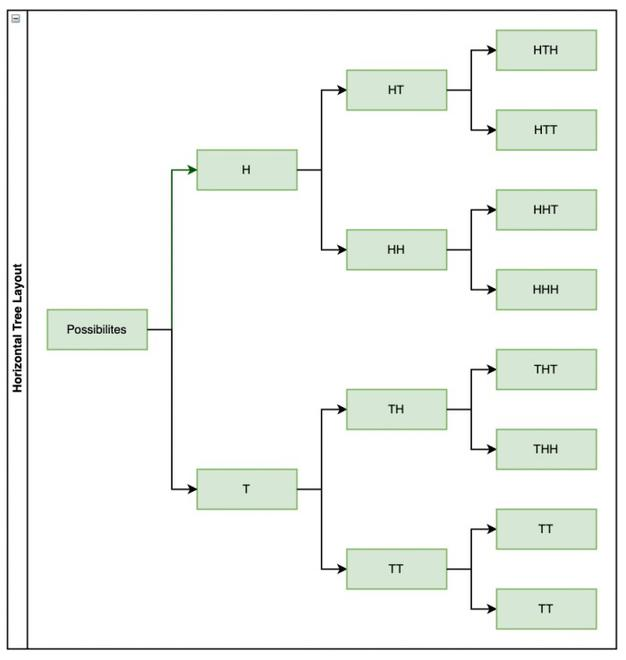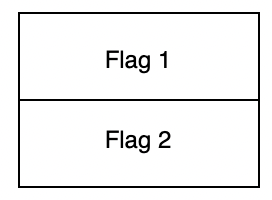Fundamental Principle of Counting

• Last Updated : 21 Feb, 2021

Let’s imagine there is a suitcase with a number lock on it, and the owner forgot his password. The number lock has 4 wheels and each one is labeled with 10 digits from 0 to 9. It can only be unlocked if 4 specific digits are arranged in a particular sequence. But the problem is the owner has forgotten that specific sequence. When asked he just remembers that the first digit is 7. In order to open the lock, how many sequences of 3-digits he may have to check with? To answer this question he immediately starts listing all possible arrangements of 9 remaining digits taken 3 at a time. But, this method will be tedious, because the number of possible sequences may be large. Let’s see some basic counting techniques which enable us to solve such problems.

Fundamental Principle of Counting

To understand this principle intuitively let’s consider an example. Let’s say a person has 3 pants and 2 shirts and a question pops up, how many different ways are there in which he can dress? There are three different ways of choosing pants as there are three types of pants available. Similarly, there are two ways of choosing shirts.

Let’s see all the different ways of dressing through a diagram. Considering P1, P2, and P3 as pants and S1, S2 as shirts. The tree given below lists the range of possibilitiesTree Diagram depicting the number of possibilities for the above example

As shown in the figure, with each type of pant. There are two possible shirts that can be worn. So in total, there are 6 ways of dressing up.

Question: Count the number of possibilities when a coin is tossed 3 times using a tree diagram.So, there are in total 8 possibilities.

But in these scenarios, it’s not possible for us to manually count the number of possibilities every time or make a tree of them. In this case, the Fundamental principle of counting helps us. It says,

“If an event can occur in m different ways, following which another event can occur in n different ways, then the total number of occurrence of the events in the given order is m×n.”

This principle can be extended to any finite number of events in the same way. So, for three events this principle becomes,

“If an event can occur in m different ways, following which another event can occur in n different ways, following both of these events another event happens which can occur in p different ways. So, then the total number of occurrence of the events in the given order is “m × n × p”.

Examples and Applications

Let’s look at examples of this principle to make our understanding concrete.

Question 1: Find the number of four-letter words with or without meaning, which can be made out of letters of the word ROSE, where the repetition of letters is not allowed.

Number of words that can be formed from these four-letter words is equal number ways in which we can fill __ __ __ __ with letters R, O, S, E. Note that repetition is not allowed. The first place can be filled with any of the four letters, after that second place can only be filled by three letters because we have already used one and repetition is not allowed. Third place can only be filled by two letters and last place will be filled with the last remaining letter.

So, number of ways in which we can do this are. 4 × 3 × 2 × 1 = 24.

Note: If the repetition of the letters was allowed we could have always used four letters to fill each place. So 4 × 4 × 4 × 4 = 256.

Question 2: Given 6 flags of different colors, how many different signals can be generated, if a signal requires the use of 2 flags one below the other?

A signal can be seen like this.Here in each position we can use the different colors of flag we are given. So, in the first position we have 6 different choices to make to fill in the position of flag 1. So, in the second position we will have 5 positions to fill because we have already used one color.

So, total number of ways to fill = 6 × 5 = 30.

Question 3: How many 2 digit even numbers can be formed from the digits 1, 2, 3, 4, 5 if the digits can be repeated?

__ __, there are five possibilities for putting numbers in each place since the numbers can be repeated. But a constraint is given in the questions which says that the number should be even. So, all the even numbers have even digit as the last digit. In the given numbers, only 2 and 4 are two even numbers. So, at the unit’s place in the number, there are only two possibilities while their 5 possibilities for the tens place.

So, total number of possible even number = 5 × 2

= 10

Question 4: How many positive divisors do 1000 = 2353 have?